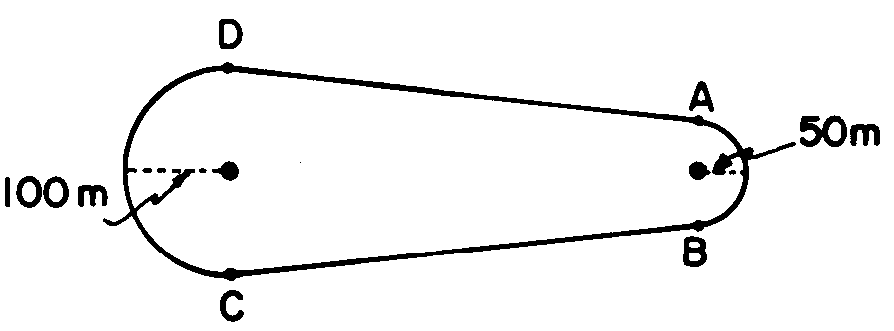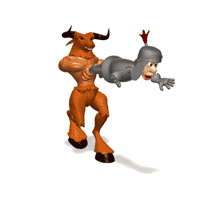A car moving in a circle is
always turning in the same direction

Penn State Schuylkill

Go Nittany Lions!!Identify the Fc and v.
What is the relationship between Fc and v?

[ Flash ]

Cut the Rope

D. Centripetal Force (Fc) - force directed toward center of circle which keeps an object moving in a uniform circular path

 Fc  = mac = mv2 r

AP Physics p. 130) 8, 11

 Ex) A 5.0 kg object moves in a circle at a constant speed of 10. m/s. What is the radius of the object's circular path if the object's centripetal force is 1000. N?Ex) A 5.0 kg object moves in a circle at a constant speed of 10. m/s. What is the radius of the object's circular path if the object's centripetal force is 1000. Newtons?

 Fc = mv 2 r

 1000. N = 5.0 kg(10. m/s)2 r

(1000.) = 500/r

1000r = 500

r = 500/(1000)

r = .50 metersImage created by John Wetzel, founding author of WikiPremed.

E. Changing Centripetal Force

1. Fc and Mass?

Put your mouse over each variable above

[ Flash ]

Direct Relationship

(Both variables on top)

 Mass Fc x 2 x 2 x 3 x 3 x 4 x 4 x 1/2 x 1/2 x 1/4 x 1/4

[ Flash ]

Inverse Relationship

(One variable on top,
other on bottom)

 Fc r x 1/2 x 2 x 1/3 x 3 x 1/4 x 4 x 2 x 1/2 x 4 x 1/4

A car moves around a track at constant speed.
When is the acceleration greatest?

What is the acceleration from A to D?a is greatest from A to B. r is small.

3. Fc & Velocity?

[ Flash ]

Direct Square Relationship(Both variables on top, one is squared)

 V Fc x 2 x 4 x 3 x 9 x 4 x 16 x 1/2 x 1/4 x 1/4 x1/16

2 Step Challenge

 Ex) A toy car moves in a circular track of radius 5.00 m in 10.0 seconds. What is the car's centripetal acceleration?

ac = V2/r

r = 5.00 m

t = 10.0 sec

a = ?

Need to first find V!!!

V = Dd/DtV = (2∏r)/t

 = [2∏(5.00 m)] (10.0 sec)

V = 3.14 m/s

ac = V2/r

ac = (3.14 m/s)2 /(5.00 m)

ac = 1.97 m/s2

[ Flash ]

Hammer ThrowTeaching from Space: Centripetal Force

`This media asset is adapted from video provided by NASA's Teaching From Space program. `
```Produced bt WNET.org

http://www.teachersdomain.org/resource/npe11.sci.phys.maf.centripetal/```